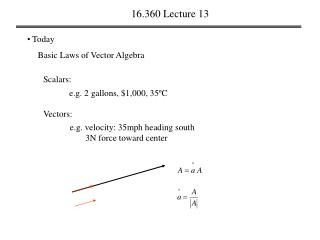DownloadDownload Presentation16.360 Lecture 13

# 16.360 Lecture 13

Download Presentation## 16.360 Lecture 13

- - - - - - - - - - - - - - - - - - - - - - - - - - - E N D - - - - - - - - - - - - - - - - - - - - - - - - - - -
##### Presentation Transcript

1. 16.360 Lecture 13 • Today Basic Laws of Vector Algebra Scalars: e.g. 2 gallons, \$1,000, 35ºC Vectors: e.g. velocity: 35mph heading south 3N force toward center

2. 16.360 Lecture 13 • Cartesian coordinate system z A  y  x

3. 16.360 Lecture 13 • Vector addition and subtraction C = B+A = A +B, A C C parallelogram rule A head-to-tail rule B B |C| = |B+A| = |A| +|B|, D = A - B = -(B –A), A A D D’ = (B-A) B B

4. 16.360 Lecture 13 • position and distance z A B y D = A - B = -(B –A), x

5. 16.360 Lecture 13 • Vector multiplication 1. simple product 2. scalar product (dot product)

6. 16.360 Lecture 13 Properties of scalar product (dot product) a) commutative property b) Distributitve property

7. 16.360 Lecture 13 3. vector product (cross product) a) anticommutative property b) Distributitve property c)

8. 16.360 Lecture 13 3. vector product (cross product)

9. 16.360 Lecture 13 Example vectors and angles • In Cartesian coordinate, vector A is directed from origin to point P1(2,3,3), and vector B is directed from P1 to pint P2(1,-2,2). Find: • (a) Vector A, its magnitude |A|, and unit vector a • (b) the angle that A makes with the y-axis • (c) Vector B • (d) the angle between A and B • (e) perpendicular distance from origin to vector B

10. 16.360 Lecture 13 4. Scalar and vector triple product a) scalar triple product b) vector triple product

11. 16.360 Lecture 13 Example vector triple product